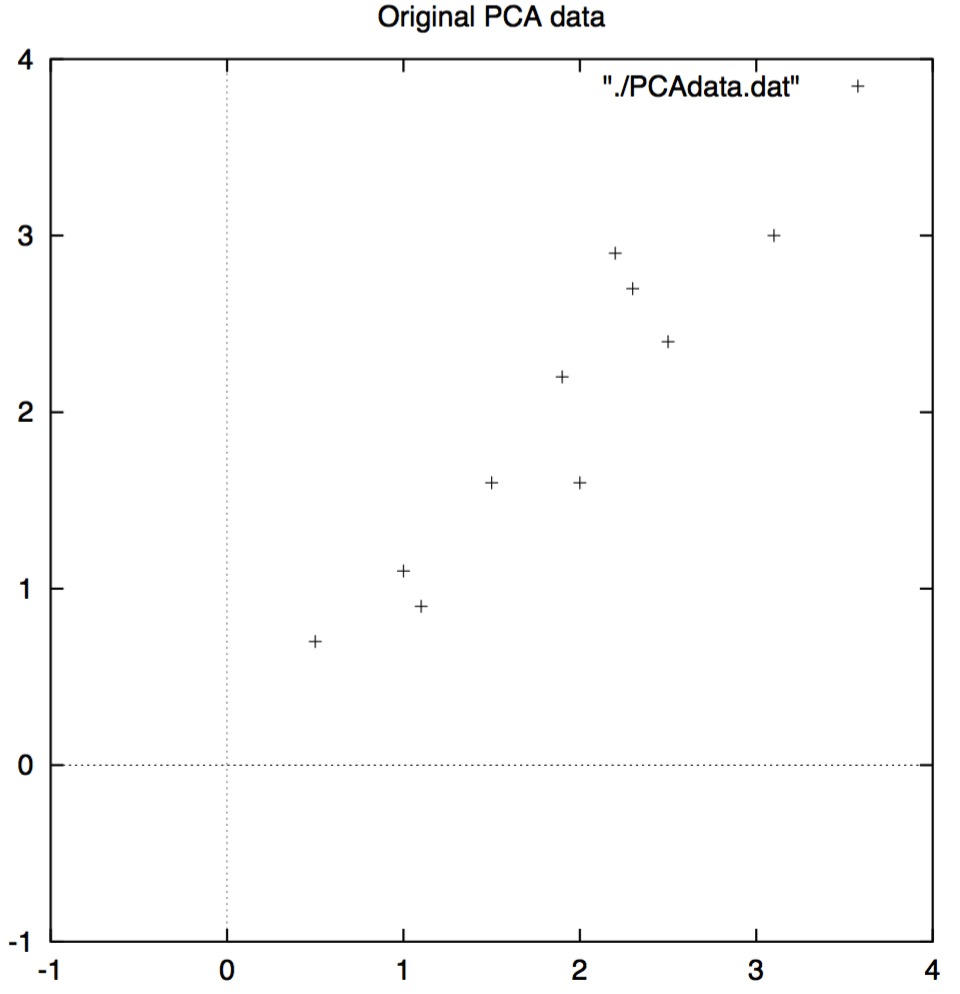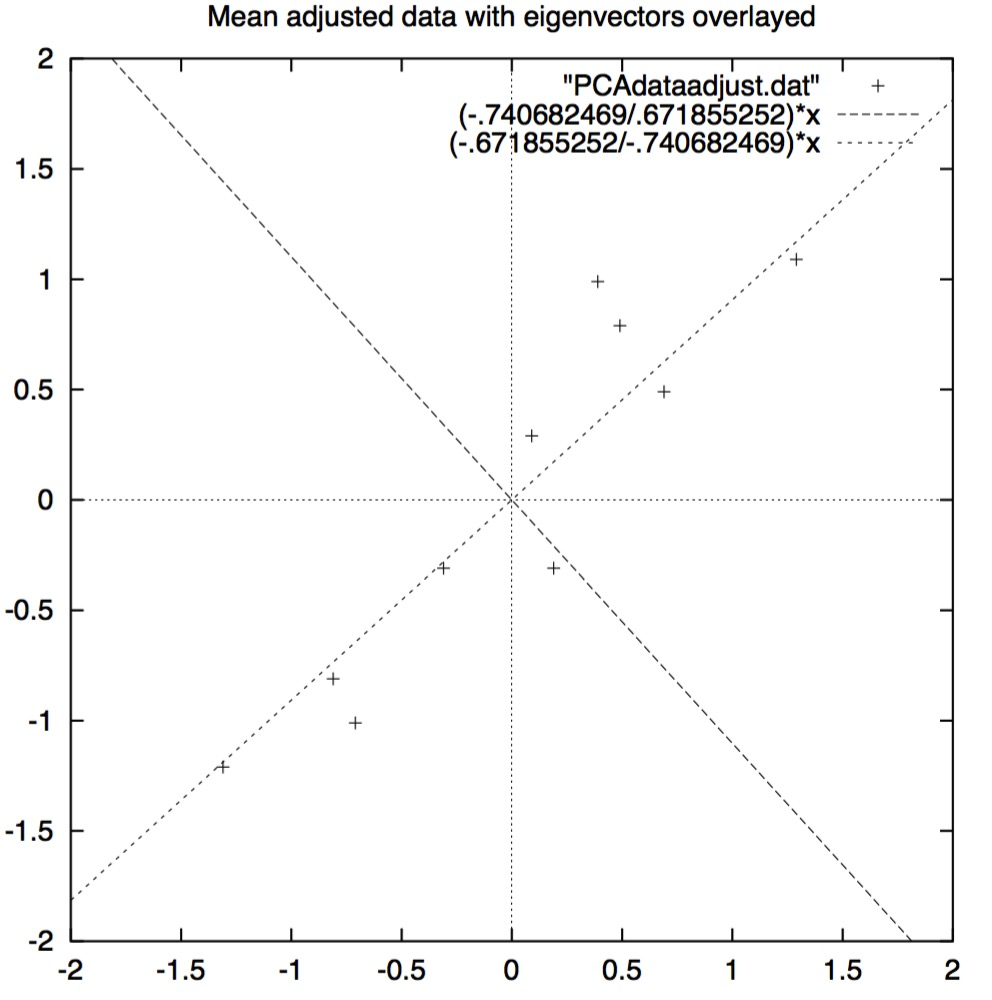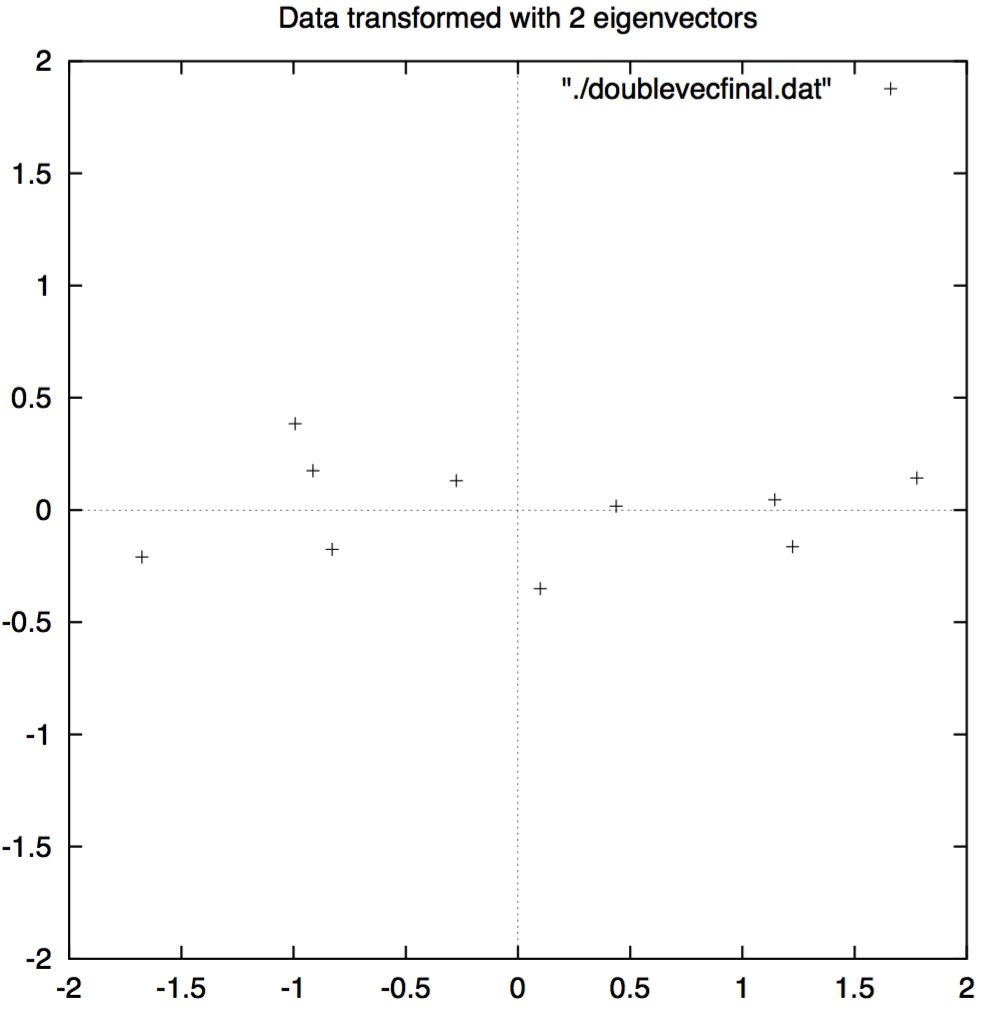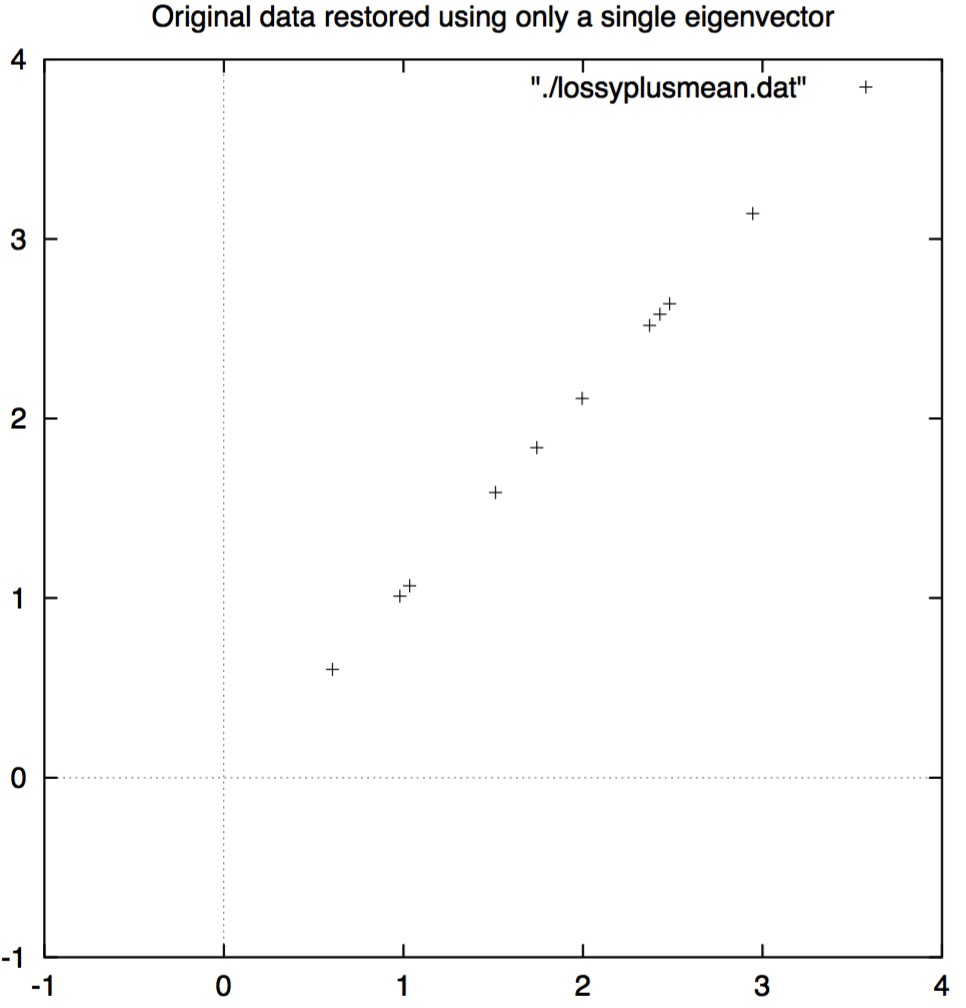### 第二章

#### 2.1 统计知识

##### 2.1.1 标准差

$$X=[1\, 2\,4\, 6\, 12\, 15\, 25\, 45\, 68\, 67\, 65\, 98]$$

$$\overline{X} =\frac{\sum_{i=1}^n X_i}{n}$$

$$[0\, 8\, 12\, 20]\quad 和 \quad[8\, 9\, 11\, 12]$$

$$s=\sqrt{\frac{\sum_{i=1}^n (X-\overline{X})^2}{(n-1)}}$$

$$\begin{array}{lrr} X & (X-\overline{X}) & (X-\overline{X})^2 \ \hline 0 & -10 & 100\ 8 & -2 & 4\ 12 & 2 & 4\ 20 & 10 & 100\ \hline \bf{总计} & & 208\ \hline \bf{除以(n-1)} & & 69.333\ \hline \bf{平方根} & & 8.3266\ \hline \end{array}$$

\begin{array}{lrr}
X & (X-\overline{X}) & (X-\overline{X})^2 \
\hline
8 & -2 & 4\
9 & -1 & 1\
11 & 1 & 1\
12 & 2 & 4\
\hline
\bf{总计} & & 10\
\hline
\bf{除以(n-1)} & & 3.333\
\hline
\bf{平方根} & & 1.8.257\
\hline
\end{array}
$$\bf{表2.1 标准差计算}$$

$$[10\, 10\, 10\, 10]$$

##### 2.1.2 方差

$$s^2=\frac{\sum_{i=1}^n (X-\overline{X})^2}{(n-1)}$$

##### 练习

[12 23 34 44 59 70 98]

[12 15 25 27 32 88 99]

[15 35 78 82 90 95 97]

##### 2.1.3 协方差

$$var(X)=\frac{\sum_{i=1}^n(X_i-\overline{X_i})(X_i-\overline{X_i})}{(n-1)}$$

$$cov(X,Y)=\frac{\sum_{i=1}^n(X_i-\overline{X_i})(Y_i-\overline{Y_i})}{(n-1)}$$

##### 2.1.4

\begin{array}{lrr}
&小时数(H)&成绩(M)\
\hline

& 15 &56 \
& 25 &93 \
& 14 &61 \
& 10 &50 \
& 18 &75 \
& 0 &32 \
& 16 &85 \
& 5 &42 \
& 19 &70 \
& 16 &66 \
& 20 &80 \
\hline

\hline

\hline
\end{array}

\begin{array}{cc|c|c|c}
H & M & (H_i - \overline{H}) & (M_i-\overline{M}) & (H_i-\overline{H})(M_i-\overline{M})\
\hline
9 & 39 & -4.92& -23.42 &115.23\
15 & 56 & 1.08& -6.42 &-6.93\
25 & 93 & 11.08& -30.58 &338.83\
14 & 61 & 0.08& -1.42 &-0.11\
10 & 50 & -3.92& -12.42 &48.69\
18 & 75 & 4.08& 12.58 &51.33\
0 & 32 & -13.92& -30.42 &423.45\
16 & 85 & 2.08& -22.58 &46.97\
5 & 42 & -8.92& -20.42 &182.15\
19 & 70 & 5.08& -7.58 &38.51\
16 & 66 & 2.08& -3.58 &7.45\
20 & 80 & 6.08& 17.58 &106.89\
\hline

\hline

\end{array}

$$C^{n\times n}=(c_{i,j}, c_{i,j}=cov(Dim_i, Dim_j))$$,

\begin{pmatrix}
cov(x,x) & cov(x,y) & cov(x,z) \
cov(y,x) & cov(y,y) & cov(y,z) \
cov(z,x) & cov(z,y) & cov(z,z) \
\end{pmatrix}

##### 练习
1. 计算以下关于$x$和$y$的2维数据集的协方差，然后描述一下协方差结果可能推导出数据什么方面的结论。
\begin{array}{c|c|c|c|c|c}
项目id & 1 & 2 & 3 & 4 & 5\
\hline
x & 10 & 39 & 19 & 23 & 28\
y & 43 & 13 & 32 & 21 & 20\
\hline
\end{array}
2. 计算下列3维数据的协方差矩阵：
\begin{array}{c|c|c|c}
项目id & 1 & 2 & 3 \
\hline
x & 1 & -1 & 4\
y & 2 & 1 & 3\
z & 1 & 3 & -1\
\hline
\end{array}

#### 2.2 矩阵代数

\begin{align}\begin{pmatrix}
2 & 3\
2 & 1\
\end{pmatrix}\times
\begin{pmatrix}
1\\
3
\end{pmatrix}=
\begin{pmatrix}
11\\
5
\end{pmatrix}
\end{align}

\begin{align}\begin{pmatrix}
2 & 3\
2 & 1\
\end{pmatrix}\times
\begin{pmatrix}
3\\
2
\end{pmatrix}=
\begin{pmatrix}
12\\
8
\end{pmatrix}=4\times
\begin{pmatrix}
3\\
2
\end{pmatrix}
\end{align}
$$\bf{图2.2：非特征向量和1个特征向量}$$
\begin{align}
2\times
\begin{pmatrix}
3\\
2
\end{pmatrix}=
\begin{pmatrix}
6\\
4
\end{pmatrix}
\end{align}

\begin{align}\begin{pmatrix}
2 & 3\
2 & 1\
\end{pmatrix}\times
\begin{pmatrix}
6\\
4
\end{pmatrix}=
\begin{pmatrix}
24\\
16
\end{pmatrix}=4\times
\begin{pmatrix}
6\\
4
\end{pmatrix}
\end{align}

$$\bf{图2.3: 缩放特征向量后仍为特征向量}$$

##### 2.2.1 特征向量

$$\begin{pmatrix} 3\ 2 \end{pmatrix}$$

$$\sqrt{(3^2+2^2)}=\sqrt{13}$$

$$\begin{pmatrix} 3\ 2 \end{pmatrix}\div\sqrt{13}= \begin{pmatrix} {3}/{\sqrt{13}}\ {2}/{\sqrt{13}} \end{pmatrix}$$

#### 练习

$$\begin{pmatrix} 3&0&-1\ -4&1&2\ -6&0&-2 \end{pmatrix}$$

$$\begin{pmatrix} 2\ 2\ -1 \end{pmatrix}\ \begin{pmatrix} -1\ 0\ 2 \end{pmatrix}\ \begin{pmatrix} -1\ 1\ 3 \end{pmatrix}\ \begin{pmatrix} 0\ 1\ 0 \end{pmatrix}\ \begin{pmatrix} 3\ 2\ 1 \end{pmatrix}$$

### 第三章 主成分分析（Principal Components Analysis）

PCA表现出色。我做的是为你提供每一步都发生了什么，这样，将来如果你想使用此技术时，就会有足够多的知识帮助你做决策。

#### 3.1 方法

##### 第二步：减掉均值

\begin{align}
\bf{数据}=
\begin{array}{c|c}
x& y\
\hline
2.5 & 2.4\
0.5 & 0.7\
2.2 & 2.9\
1.9 & 2.2\
3.1 & 3.0\
2.3 & 2.7\
2 & 1.6\
1 & 1.1\
1.5 & 1.6\
1.1 & 0.9\
\end{array}\bf{调整后的数据=}
\begin{array}{c|c}
x& y\
\hline
0.69 & 0.49\
-1.31 & -1.21\
0.39 & 0.99\
0.09 & 0.29\
1.29 & 1.09\
0.49 & 0.79\
0.19 & -0.31\
-0.81 & -0.81\
-0.31 & -0.31\
-0.71 & -1.01\
\end{array}
\end{align}$$图3.1：PCA示例数据，左边为原始数据，右边为减掉均值的数据$$

##### 第三步：计算协方差矩阵

$$cov=\begin{pmatrix} 0.616555556&0.615444444\ 0.615444444&0.716555556 \end{pmatrix}$$

##### 第四步：计算协方差矩阵的特征向量和特征值

\begin{align}
\bf{特征值}=\begin{pmatrix}
0.0490833989\
1.28402771
\end{pmatrix}\bf{\bf 特征向量}=\begin{pmatrix}
-0.7351178656 & -0.6778873399\
0.677873399 & -0.735178656
\end{pmatrix}
\end{align}

##### 第五步：选择成分及构建特征的向量$$图3.2:\bf{标准化（减掉均值）后的数据图以及协方差矩阵中特征向量图}$$

$$\bf{特征的向量}=(eig_1,eig_2,eig_3,…,eig_p)$$

$$cov=\begin{pmatrix} -0.77873399&-0.735178656\ -0.735178656&0.677873399 \end{pmatrix}$$

$$cov=\begin{pmatrix} -0.677873399\ -0.735178656 \end{pmatrix}$$

##### 第六步：生成新数据集

$$\bf{最终数据=行特征的向量} \times \bf{行调整后的数据}$$

##### 3.1.1 把旧数据找回来

\begin{align}
\bf{转换后的数据}=\begin{array}{c|c}
x&y\
\hline
-0.827970186 & -0.175115307\
1.77758033 & 0.142857227\
-0.992197494 & 0.384374989\
-0.274210416 & 0.1304117207\
-1.67580142 & -0.209498461\
-0.912949103 & 0.17528282444\
0.0991094375 & -0.349824698\
1.14457216 & 0.0464172582\
0.438046137 & 0.0177646297\
1.22382056 & -0.162675287\
\end{array}
\end{align}$$图3.3：应用了PCA分析后并使用了两个特征向量的数据表以及绘制的新数据点$$

\begin{array}{c}
x\
\hline
-0.827970186\
1.77758033\
-0.992197494\
-0.274210416\
-1.67580142\
-0.912949103\
1.14457216\
0.438046137\
1.22382056
\end{array}
$$图3.4：只用最重要的特征向量数据转换的数据$$

$$\bf{最终数据=行特征的向量} \times \bf{行调整后的数据}$$

$$\bf{行调整后的数据}=\bf{行特征的向量}^{-1}\times\bf{最终数据}$$

$$\bf{行调整后的数据}=\bf{行特征的向量}^{T}\times\bf{最终数据}$$$$图3.5 从单独一维特征向量重新构造的数据$$

#### 练习

1. 协方差矩阵的特征向量为我们提供了什么呢？
2. 我们在PCA计算的过程中，哪一步可以决定压缩数据，压缩可以起到什么效果呢？
3. 举例说明PCA在图像处理怎样用主成分表示，同时调研一下人脸识别中“特征脸”(Eigenfaces)主题。

### 第四章 计算机视觉应用

#### 4.1 表示

$$X=(x_1,x_2,x_3,…,x_{N^2})$$

#### 4.2 PCA寻找模式

$$图像矩阵= \begin{pmatrix} ImageVec_1\ ImageVec_2\ \vdots\ ImageVec_{20}\ \end{pmatrix}$$